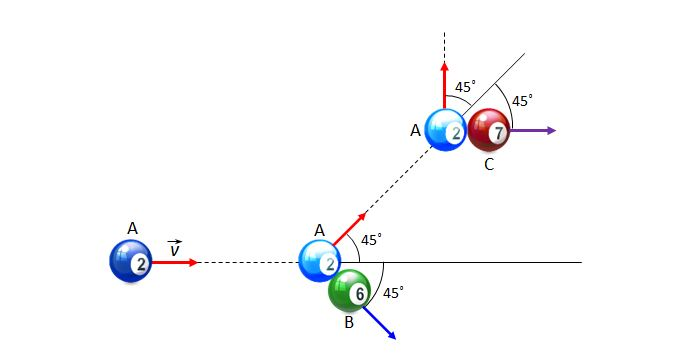# Three-cushion billiardsOn a frictionless plane, ball $A$ moves with a velocity of $v$ to collide elastically with two stopped balls $B$ and $C$ sequentially, as shown above. The masses of the three balls are the same as $m.$ As indicated by the red arrows, the direction of ball $A$'s movement changes by an angle of $45$° each time there is a collision. What is the sum of the quantities of the impulses acting on ball $A$ by balls $B$ and $C?$

×